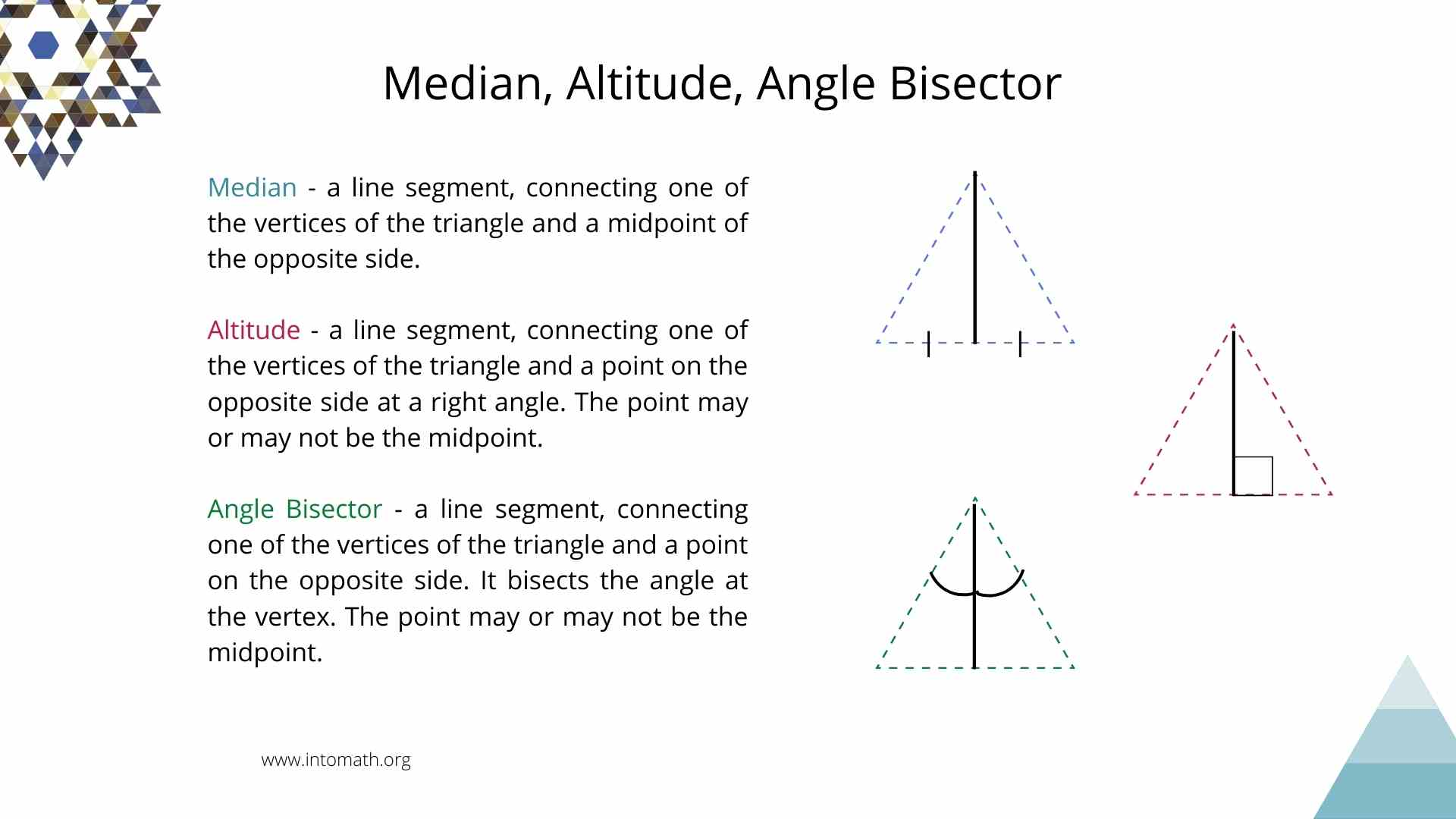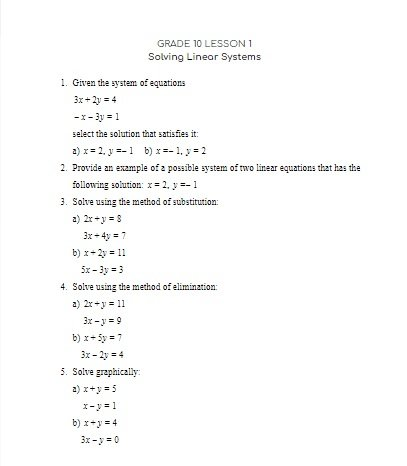CA\$2.00

In this lesson we are learning about a triangle and triangle properties. You have been working with triangles for a while now. You may already know that there are different types of triangles: equilateral, isosceles and scalene. There are right (one of the angles is 90o) and non-right angle triangles).

Triangles are congruent if they completely overlap when placed on top of each other. This means that their corresponding sides and angles are equal.

There is a number of important triangle properties.

We label the sides using lower case letters and the angles using upper case letters and the side opposite the angle is labelled with the same letter (E-g: a and A).

For example, that all interior angles inside a triangle add up to 180o.

A Midpoint is a point in the middle of the side of the triangle.

Midpoint Segment is a line segment, connecting two midpoints. It is parallel to the opposite side of the triangle and it half of its length.

Median – a line segment, connecting one of the vertices of the triangle and a midpoint of the opposite side.

Altitude – a line segment, connecting one of the vertices of the triangle and a point on the opposite side at a right angle. The point may or may not be the midpoint.